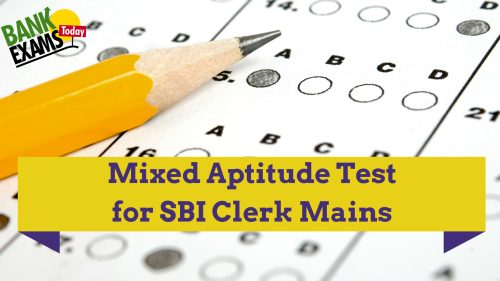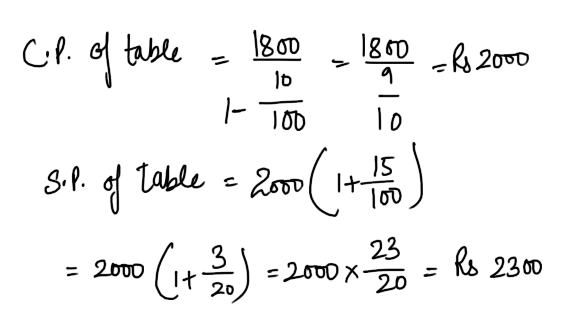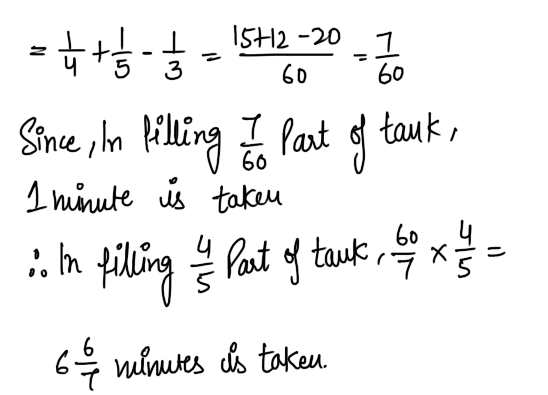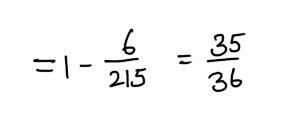# Mixed Aptitude Quiz for SBI Clerk Mains (Part - 13)#### Ques 1.

A table was sold for Rs. 1,800 at a loss of 10% For What it should have been sold to earn a profit of 15% ?
(a) Rs. 2,070
(b) Rs. 1,890
(c) Rs. 2,000
(d) None of these
Ans 1.#### Ques 2.

405 sweets were distributed equally among children in such a way that each child got sweets equivalent of 20% of the total children.How many sweets did each child get ?
(a) 15
(b) 45
(c) 9
(d) 18
Ans 2.  Suppose  each child got x items
. . 405/x = 20%  of  x =>  x = 45.

#### Ques 3.

A tank is filled up by pipe A and B in4 and 5 minutes separately but pipe C can empty it in 3 minutes. if all the three pipes can be opened the 4/5 part of tank can be either filled or emptied in
(a) 1 5/7 minutes in filling
(b) 1 6/7 minutes in filling
(c) 1 5/7 minutes in empty
(d) None of the above
Ans 3. If all the three pipes are opened then the tank will be filled. Now filled  part of tank in 1 minute#### Ques 4.

what is the least number which when divided by the numbers 3,5,6,8,10 and 12, leaves in each case a remainder 2 but which when divided by 13 leaves no remainder ?
(a) 312
(b) 962
(c) 1562
(d) 1586
Ans 4.  L.C.M. pf 3, 5, 6, 8,  and 10 = 120
. . The number 122 leaves a remainder of 2 when divided by 3, 5, 6, 8, and 10.
Least multiple of 120 + 2 , i.e. , 120 x 8 + 2 = 960 +2 = 962, when  divided by 13, leaves a remainder of zero.

#### Ques 5.

A can finish a work in 18 days and B can do the same work in 15 days. B worked for 10 days and left the job. In how many days. A alone can finish the remaining work ?
(a) 5
(b) 5 1/2
(c) 6
(d) 8
Ans 5.#### Ques 6.

A refrigerator is offered for sale at Rs.10,000 less successive discounts of 20% and 15%. The sale price of the refrigerator is
(a) 35% less than Rs. 10,000
(b) 68% of Rs. 10,000
(c) 65% of Rs. 10,000
(d) 77% of Rs. 10,000
Ans 6.#### Ques 7.

The average age of the boys in a class is 16 years and that of the girls is 15 years. The average age for the whole class is
(a) 15.5 Years
(b) 16 years'
(c) 15 years

#### Ques 8.

A crown made of old, silver,coper and brass  weighs 9.725 kg. The weight  of the gold and silver together is 4 kg and the weight of the gold and copper 4.5kg and  of the gold and brass 3.6 kg . what is the weight of the gold in the crown ?
(a) 1.2500 kg
(b) 2.6576 kg
(c) 1.1875 kg
(d) 2.3705 kg
Ans 8. For an income of Rs 12, stock needed =Rs. 100
For an income  of Rs. 1200 , stock needed#### Ques 9.

In a single throw of three dice, what is the probability of not getting the same number on all the dice ?
(a) 35/36
(b) 36/35
(c) 1/36
(d) None of these
Ans 9. n (S) = 216
Same number on three dice : 111, 222, 333, 444, 555, 666.
P( not getting same number on all dice = 1-P ( getting same number on all dice)#### Ques 10.

Two pipes can fill a tank in 12 hours and 15 hours respectively . A third pipe can empty it in 20 hours . If the tank is empty and all the three pipes are opened, then the tank will be full in
(a) 7 hours
(b) 9 hours
(c) 10 hours
(d) 14 hours
Ans 10.#### Ques 11.

Saturday was a holiday for a Republic Day. 14th of the next month is again
a holiday for Shivratri. What day was it on the 14th ?
(a) Thursday
(b) Friday
(c) Saturday
(d) Monday
Ans 11.  Thursday

#### Ques 12.

In 4 years, the simple interest on a certain sum of money is 9/25 of the principal. The annual rate of interest is
(a) 4%
(b) 4 1/2 %
(c) 9%
(d) 10%
Ans 12. Let the sum be Rs .x
let the rate be R%